# 54 Results

View
Selected filters:
• Equations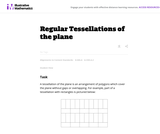Unrestricted Use
CC BY
Rating

This task examines the ways in which the plane can be covered by regular polygons in a very strict arrangement called a regular tessellation. These tessellations are studied here using algebra, which enters the picture via the formula for the measure of the interior angles of a regular polygon (which should therefore be introduced or reviewed before beginning the task). The goal of the task is to use algebra in order to understand which tessellations of the plane with regular polygons are possible.

Subject:
Mathematics
Algebra
Geometry
Material Type:
Activity/Lab
Provider:
Illustrative Mathematics
Provider Set:
Illustrative Mathematics
Author:
Illustrative Mathematics
01/21/2013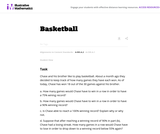Unrestricted Use
CC BY
Rating

This task provides a simple but interesting and realistic context in which students are led to set up a rational equation (and a rational inequality) in one variable, and then solve that equation/inequality for an unknown variable.

Subject:
Mathematics
Algebra
Material Type:
Activity/Lab
Provider:
Illustrative Mathematics
Provider Set:
Illustrative Mathematics
Author:
Illustrative Mathematics
12/15/2012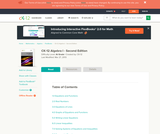Conditional Remix & Share Permitted
CC BY-NC-SA
Rating

A work in progress, CK-12's Algebra I Second Edition is a clear presentation of algebra for the high school student. Topics include: Equations and Functions, Real Numbers, Equations of Lines, Solving Systems of Equations and Quadratic Equations.

Subject:
Algebra
Functions
Material Type:
Textbook
Provider:
CK-12 Foundation
Provider Set:
CK-12 FlexBook
Author:
Andrew
Annamaria
Anne
Eve
Farbizio
Gloag
Rawley
12/03/2010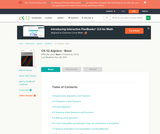Conditional Remix & Share Permitted
CC BY-NC-SA
Rating

CK-12 Foundation's Basic Algebra FlexBook is an introduction to the algebraic topics of functions, equations, and graphs for middle-school and high-school students.

Subject:
Algebra
Functions
Material Type:
Textbook
Provider:
CK-12 Foundation
Provider Set:
CK-12 FlexBook
Author:
Farbizio, Annamaria
Gloag, Andrew
Gloag, Anne
Kramer, Melissa
09/21/2010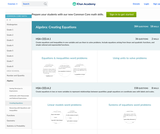Conditional Remix & Share Permitted
CC BY-NC-SA
Rating

This site teaches High Schoolers how to create equations through a series of 298 questions and interactive activities aligned to 5 Common Core mathematics skills.

Subject:
Mathematics
Algebra
Material Type:
Activity/Lab
Interactive
Provider:
Provider Set:
11/17/2020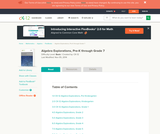Conditional Remix & Share Permitted
CC BY-NC-SA
Rating

CK-12 Algebra Explorations is a hands-on series of activities that guides students from Pre-K to Grade 7 through algebraic concepts.

Subject:
Algebra
Material Type:
Activity/Lab
Teaching/Learning Strategy
Provider:
CK-12 Foundation
Provider Set:
CK-12 FlexBook
Author:
Carol ­ Findell, Mary ­Cavanagh, Carole ­Greenes
02/23/2012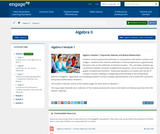Conditional Remix & Share Permitted
CC BY-NC-SA
Rating

Students connect polynomial arithmetic to computations with whole numbers and integers.  Students learn that the arithmetic of rational expressions is governed by the same rules as the arithmetic of rational numbers.  This unit helps students see connections between solutions to polynomial equations, zeros of polynomials, and graphs of polynomial functions.  Polynomial equations are solved over the set of complex numbers, leading to a beginning understanding of the fundamental theorem of algebra.  Application and modeling problems connect multiple representations and include both real world and purely mathematical situations.

Subject:
Algebra
Material Type:
Module
Provider:
New York State Education Department
Provider Set:
EngageNY
05/14/2013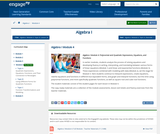Conditional Remix & Share Permitted
CC BY-NC-SA
Rating

In earlier modules, students analyze the process of solving equations and developing fluency in writing, interpreting, and translating between various forms of linear equations (Module 1) and linear and exponential functions (Module 3). These experiences combined with modeling with data (Module 2), set the stage for Module 4. Here students continue to interpret expressions, create equations, rewrite equations and functions in different but equivalent forms, and graph and interpret functions, but this time using polynomial functions, and more specifically quadratic functions, as well as square root and cube root functions.

Subject:
Algebra
Material Type:
Module
Provider:
New York State Education Department
Provider Set:
EngageNY
09/17/2013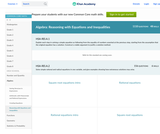Conditional Remix & Share Permitted
CC BY-NC-SA
Rating

This site teaches Reasoning with Equations and Inequalities to High Schoolers through a series of 5909 questions and interactive activities aligned to 36 Common Core mathematics skills.

Subject:
Mathematics
Algebra
Material Type:
Activity/Lab
Interactive
Provider:
Provider Set:
11/17/2020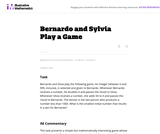Unrestricted Use
CC BY
Rating

This task presents a simple but mathematically interesting game whose solution is a challenging exercise in creating and reasoning with algebraic inequalities. The core of the task involves converting a verbal statement into a mathematical inequality in a context in which the inequality is not obviously presented, and then repeatedly using the inequality to deduce information about the structure of the game.

Subject:
Mathematics
Algebra
Material Type:
Activity/Lab
Provider:
Illustrative Mathematics
Provider Set:
Illustrative Mathematics
Author:
Illustrative Mathematics
10/21/2013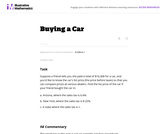Unrestricted Use
CC BY
Rating

The emphasis in this task is on the progression of equations, from two that involve different values of the sales tax, to one that involves the sales tax as a parameter. It is designed to foster the habit of looking for regularity in solution procedures, so that students don't approach every equation as a new problem but learn to notice familiar types.

Subject:
Mathematics
Algebra
Material Type:
Activity/Lab
Provider:
Illustrative Mathematics
Provider Set:
Illustrative Mathematics
Author:
Illustrative Mathematics
05/01/2012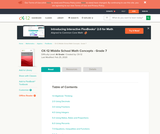Conditional Remix & Share Permitted
CC BY-NC-SA
Rating

CK-12 Middle School Math Concepts for seventh grade provides a complete textbook. It presents topics including algebraic thinking, patterns, decimals, decimal operations, fractions, fraction operations, integers, integer operations, ratios, rates, proportions, percents, percent applications, equations, solving equations, inequalities, functions, graphing functions, geometry, plane geometry, solid geometry, area, perimeter, surface area, volume, statistics including mean, median, mode and range, graphing and types of graphs, and probability.

Subject:
Mathematics
Algebra
Geometry
Statistics and Probability
Material Type:
Textbook
Provider:
CK-12 Foundation
Provider Set:
CK-12 FlexBook
Author:
Jen Kershaw
11/30/2012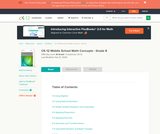Conditional Remix & Share Permitted
CC BY-NC
Rating

In CK-12 Middle School Math Concepts – Grade 8, the learning content is divided into concepts. Each concept is complete and whole providing focused learning on an indicated objective. Theme-based concepts provide students with experiences that integrate the content of each concept. Students are given opportunities to practice the skills of each concept through real-world situations, examples, guided practice and explore more practice. There are also video links provided to give students an audio/visual way of connecting with the content.

Subject:
Mathematics
Material Type:
Full Course
Lesson Plan
Textbook
Unit of Study
Provider:
CK-12 Foundation
Provider Set:
CK-12 FlexBook
11/18/2020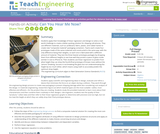Read the Fine Print
Educational Use
Rating

Students apply their knowledge of linear regression and design to solve a real-world challenge to create a better packing solution for shipping cell phones. They use different materials, such as cardboard, fabric, plastic, and rubber bands to create new “composite material” packaging containers. Teams each create four prototypes made of the same materials and constructed in the same way, with the only difference being their weights, so each one is fabricated with a different amount of material. They test the three heavier prototype packages by dropping them from different heights to see how well they protect a piece of glass inside (similar in size to iPhone 6). Then students use linear regression to predict from what height they can drop the fourth/final prototype of known mass without the “phone” breaking. Success is not breaking the glass but not underestimating the height by too much either, which means using math to accurately predict the optimum drop height.

Subject:
Algebra
Physics
Material Type:
Activity/Lab
Provider:
TeachEngineering
Provider Set:
Activities
Author:
Abbie Morneault
Brett Doudican
Kellee Callahan
08/02/2017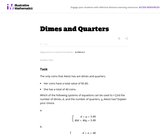Unrestricted Use
CC BY
Rating

This task does not actually require that the student solve the system but that they recognize the pairs of linear equations in two variables that would be used to solve the system. This is an important step in the process of solving systems.

Subject:
Mathematics
Algebra
Material Type:
Activity/Lab
Provider:
Illustrative Mathematics
Provider Set:
Illustrative Mathematics
Author:
Illustrative Mathematics
05/01/2012Read the Fine Print
Rating

Students create math stories by first drawing, then writing, and finally using math symbols to show addition or subtraction.

Subject:
Arts
Language, Grammar, and Vocabulary
Material Type:
Activity/Lab
Lesson Plan
Provider:
Provider Set:
11/18/2020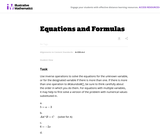Unrestricted Use
CC BY
Rating

This task asks students to use inverse operations to solve the equations for the unknown variable, or for the designated variable if there is more than one. Two of the equations are of physical significance and are examples of Ohm's Law and Newton's Law of Universal Gravitation.

Subject:
Mathematics
Algebra
Material Type:
Activity/Lab
Provider:
Illustrative Mathematics
Provider Set:
Illustrative Mathematics
Author:
Illustrative Mathematics
05/01/2012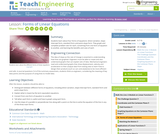Read the Fine Print
Educational Use
Rating

Students learn about four forms of equations: direct variation, slope-intercept form, standard form and point-slope form. They graph and complete problem sets for each, converting from one form of equation to another, and learning the benefits and uses of each.

Subject:
Mathematics
Algebra
Engineering
Material Type:
Lesson Plan
Provider:
TeachEngineering
Provider Set:
TeachEngineering
Author:
Aubrey McKelvey
09/18/2014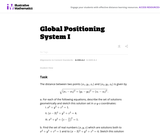Unrestricted Use
CC BY
Rating

This question examines the algebraic equations for three different spheres. The intersections of each pair of spheres are then studied, both using the equations and thinking about the geometry of the spheres. For two spheres where one is not contained inside of the other there are three possibilities for how they intersect.

Subject:
Mathematics
Algebra
Geometry
Material Type:
Activity/Lab
Provider:
Illustrative Mathematics
Provider Set:
Illustrative Mathematics
Author:
Illustrative Mathematics
01/21/2013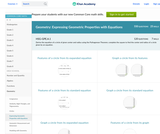Conditional Remix & Share Permitted
CC BY-NC-SA
Rating

This site teaches High Schoolers how to express geometric properties with equations through a series of 1721 questions and interactive activities aligned to 12 Common Core mathematics skills.

Subject:
Mathematics
Geometry
Material Type:
Activity/Lab
Interactive
Provider: